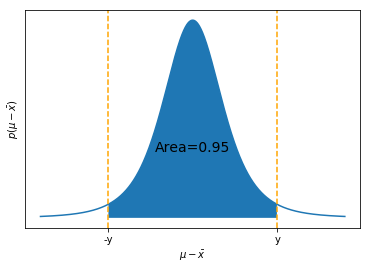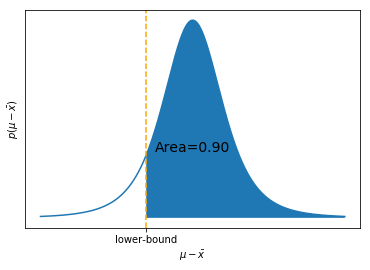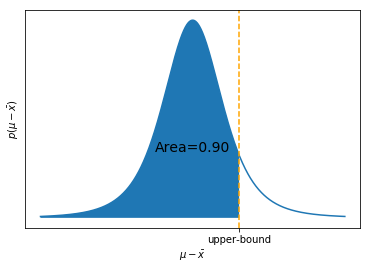# Confidence Intervals¶

## Unit 8, Lecture 2¶

Numerical Methods and Statistics

Bulmer: Pages 165-167

# Computing Confidence Interval for error in population mean with $t$-Distribution ¶

We know that the error in the population mean follows a $t$-distribution for small $N$. What if we want a confidence interval for where the true mean lies?

$$P(a < \mu < b) = 0.95$$

One simplification we can make right away is that we know $\mu$ will be centered at $\bar{x}$ and is symmetric:

$$P( \bar{x} - y < \mu< \bar{x} + y) = 0.95$$

where $y$ is some number we need to find. We can further rewrite this as:

$$P( - y < \mu - \bar{x}< + y) = 0.95$$

which we know follows a $t-$distribution. Note that these are probailities, which are integrals of the probability distribution

Here's a visual to understand what we're after. Note that I'm actually answering this problem to make the graph, so wait until later to try to understand the code!

In :
import scipy.stats as ss
import numpy as np
import matplotlib.pyplot as plt
%matplotlib inline

#make some points for plot
N = 5
x = np.linspace(-5,5, 1000)
T = ss.t.ppf(0.975, df=N-1)
y = ss.t.pdf(x, df=N-1)
plt.plot(x,y)
plt.fill_between(x, y, where= np.abs(x) < T)
plt.text(0,np.max(y) / 3, 'Area=0.95', fontdict={'size':14}, horizontalalignment='center')
plt.axvline(T, linestyle='--', color='orange')
plt.axvline(-T, linestyle='--', color='orange')
plt.xticks([-T, T], ['-y', 'y'])
plt.yticks([])
plt.ylabel(r'$p(\mu - \bar{x})$')
plt.xlabel(r'$\mu - \bar{x}$')
plt.show()This is a very similar problem to the prediction intervals we had before. We know that $p(\mu - \bar{x})$ follows a $T(0, \sigma_x /\sqrt{N}, N - 1)$ distribution and we can use the same idea as $Z$-scores as we did for prediction intervals

$$T(y) = \frac{y - 0}{\sigma_x / \sqrt{N}}$$

The 'mean' our error in the population mean distribution is 0, because our error in population mean is always centered around 0.

After taking 5 samples, we've found that the sample mean is 45 and the sample standard deviation, $\sigma_x$ is 3. What is the 95% confidence interval for the true mean, $\mu$?

We can write this more like this:

$$P(- y < \mu - \bar{x} < +y) = 0.95$$

Our interval will go from 2.5% to 97.5% (95% of probability), so let's find the $T$-value for $-\infty$ to 2.5% and 97.5% to $\infty$. Remember that the $T$-value depends on the degrees of freedom, N-1.

In :
import scipy.stats

#The lower T Value. YOU MUST GIVE THE SAMPLE NUMBER
print(scipy.stats.t.ppf(0.025, 4))
print(scipy.stats.t.ppf(0.975, 4))

-2.7764451051977996
2.7764451051977987

$$T_{low} = \frac{-y - 0}{\sigma_x / \sqrt{N}}$$$$T_{low} = -\frac{y}{\sigma_x / \sqrt{N}}$$
$$y = -T_{low}\frac{\sigma_x}{\sqrt{N}}$$
In :
print(-scipy.stats.t.ppf(0.025, 4) * 3 / np.sqrt(5))

3.7249919946113006


The final answer is $P(45 - 3.72 < 45 < 45 + 3.72) = 0.95$ or $45\pm 3.72$

# Computing Confidence Interval for Error in Population Mean Steps¶

1. Is the sample size greater than 25 OR do you know the true (population) standard deviation? If so, then use standard normal ($Z$) otherwise the $t$-distribution for your sample size ($T$).
2. Build your interval in probability. For example, a 95% double-sided goes from 2.5% to 97.5%
3. Find the $Z$ or $T$ values that match your interval. For example, $Z_{low} = -1.96$ to $Z_{high} = 1.96$ is for a double-sided 95% confidence inerval. Use the scipy.stats.t.ppf or scipy.stats.norm.ppf function to find them.
4. Use the $y = Z \sigma / \sqrt{N}$ or $y = T \sigma_x / \sqrt{N}$ equation to find the interval values in your particular distribution, where $y$ is the interval width
5. Report your answer either as an interval or the $\bar{x} \pm y$ notation.

# Shortcut Method For Normal¶

Here's how to quickly do these steps in Python for sample size greater than 25

In :
# DO NOT COPY, JUST GENERATING DATA FOR EXAMPLE
data = scipy.stats.norm.rvs(size=100, scale=15, loc=50)

In :
#Check if sample size is big enough.
#This code will cause an error if it's not
assert len(data) > 25

CI = 0.95
sample_mean = np.mean(data)
#The second argument specifies what the denominator should be (N - x),
#where x is 1 in this case
sample_var = np.var(data, ddof=1)
Z = scipy.stats.norm.ppf((1 - CI) / 2)
y = -Z * np.sqrt(sample_var / len(data))

print('{} +/ {}'.format(sample_mean, y))


51.15171700183236 +/ 2.6078997696784336


Is that low? Well, remember that our error in the mean follows standard deviation divided by the root of number of samples.

# Shortcut Method For $t$-Distribution¶

Here's how to quickly do these steps in Python for sample size less than 25

In :
# DO NOT COPY, THIS JUST GENERATES DATA FOR EXAMPLE
data = scipy.stats.norm.rvs(size=4, scale=15, loc=50)

In :
CI = 0.95
sample_mean = np.mean(data)
sample_var = np.var(data, ddof=1)
T = scipy.stats.t.ppf((1 - CI) / 2, df=len(data)-1)
y = -T * np.sqrt(sample_var / len(data))

print('{} +/ {}'.format(sample_mean, y))


50.38338953766582 +/ 36.784888003627046


# Example of Prediction Intervals¶

I know that the thickness of a metal slab is distributed according to ${\cal N}(3.4, 0.75)$. Construct a prediction interval so that a randomly chosen metal slab will lie within it 95% confidence.

$$P( \mu - y < x < \mu + y) = 0.95$$

This is a prediction interval, so we're computing a interval on the distribution itself and we know everything about it.

$$Z(\mu + y) = \frac{\mu + y - \mu}{\sigma} \Rightarrow y = \sigma Z$$$$Z = 1.96$$$$x = \mu \pm 1.96 \sigma = 3.4 \pm 1.40$$

A randomly chosen slab will have a thickness of $3.4 \pm 1.40$ 95% of the time.

# Example 1 of error in population mean with known $\sigma$¶

I measure the thickness of 35 metal slabs and find that $\bar{x}$, the sample mean, is 3.38. If I know that $\sigma = 0.75$, construct a confidence interval that will contain the true mean $\mu$ with 95% confidence.

We know that $p(\bar{x} - \mu)$ is normally distributed with ${\cal N}(0, \sigma / \sqrt{N})$. We want to find

$$P(-y < \bar{x} - \mu < +y) = 0.95$$
$$Z(+y) = \frac{y - 0}{\sigma_e} = \frac{y}{\sigma / \sqrt{N}} \Rightarrow y = \frac{\sigma}{\sqrt{N}} Z$$$$y = \frac{0.75}{\sqrt{35}}1.96 = 0.248$$$$\mu - \bar{x} = 0 \pm 0.248$$$$\mu = 3.38 \pm 0.248$$

At a 95% confidence level, the true mean is $3.38 \pm 0.248$.

# Example 2 of error in population mean with known $\sigma$¶

I measure the thickness of 11 metal slabs and find that $\bar{x}$, the sample mean, is 5.64. If I know that $\sigma = 1.2$, construct a confidence interval that will contain the true mean $\mu$ with 99% confidence.

Again we know that $p(\bar{x} - \mu)$ is normally distributed with ${\cal N}(0, \sigma / \sqrt{N})$. We want to find

$$P(-y < \bar{x} - \mu < +y) = 0.99$$
$$Z(+y) = \frac{y - 0}{\sigma_e} = \frac{y}{\sigma / \sqrt{N}} \Rightarrow y = \frac{\sigma}{\sqrt{N}} Z$$$$y = \frac{1.2}{\sqrt{11}}2.575 = 0.932$$$$\mu - \bar{x} = 0 \pm 0.932$$$$\mu = 5.64 \pm 0.932$$

# Example 1 of error in population mean with unknown $\sigma$¶

I measure the thickness of 6 metal slabs and find that $\bar{x}$, the sample mean, is 3.65 and the sample standard deviation is $1.25$. Construct a confidence interval that will contain the true mean $\mu$ with 90% confidence.

$$T(+y) = \frac{y - 0}{\sigma_x / \sqrt{N}} \Rightarrow y = \frac{\sigma_x}{\sqrt{N}} T$$

We know that $p(\bar{x} - \mu)$ is a $t$-distribution because $N$ is small. It is distributed as $T(0, \sigma_x / \sqrt{N})$. We want to find

$$P(-y < \bar{x} - \mu < +y) = 0.90$$
In :
#Notice it is 95%, so the interval goes from
#5% to 95% containing 90% of probability
T = scipy.stats.t.ppf(0.95, df=6-1)
print(T)

2.015048372669157

$$y = \frac{1.25}{\sqrt{6}} 2.015 = 1.028$$$$\mu = 3.65 \pm 1.028$$

The population mean of the slabs is $3.65 \pm 1.028$ with 90% confidence.

# Example 2 of error in population mean with unknown $\sigma$¶

I measure the thickness of 25 metal slabs and find that $\bar{x}$, the sample mean, is 3.42 and the sample standard deviation is 0.85. Construct a confidence interval that will contain the true mean $\mu$ with 90% confidence.

We know, just like last example, that $P(\bar{x} - \mu)$ is a normal distribution because $N$ is large enough for the central limit theorem to apply. It is distributed as ${\cal N}(0, \sigma_x / \sqrt{N})$. We want to find

$$P(-y < \bar{x} - \mu < +y) = 0.90$$
$$Z(+y) = \frac{y - 0}{\sigma_x / \sqrt{N}} \Rightarrow y = \frac{\sigma_x}{\sqrt{N}} Z$$$$y = \frac{0.85}{\sqrt{25}} 1.645 = 0.28$$$$\mu = 3.42 \pm 0.28$$

# Single-Sided Confidence Intervals¶

Sometimes, you would desire only to bound the population mean to be on one side.

## Upper Interval (Lower-Bound)¶

An upper-interval covers the upper x% of the probability mass and can be defined as an interval from $(y, \infty)$, where $y$ acts as a lower-bound. A visual is shown below for an upper 90% confidence interval.

In :
#make some points for plot
N = 5
x = np.linspace(-5,5, 1000)
T = ss.t.ppf(0.10, df=N-1)
y = ss.t.pdf(x, df=N-1)
plt.plot(x,y)
plt.fill_between(x, y, where= x > T)
plt.text(0,np.max(y) / 3, 'Area=0.90', fontdict={'size':14}, horizontalalignment='center')
plt.axvline(T, linestyle='--', color='orange')
plt.xticks([T], ['lower-bound'])
plt.yticks([])
plt.ylabel(r'$p(\mu - \bar{x})$')
plt.xlabel(r'$\mu - \bar{x}$')
plt.show()## Lower Interval (Upper-Bound)¶

A lower interval covers the lower x% of probability mass. It is defined with an upper bound like so: $(-\infty, y)$. An example is below:

In :
#make some points for plot
N = 5
x = np.linspace(-5,5, 1000)
T = ss.t.ppf(0.90, df=N-1)
y = ss.t.pdf(x, df=N-1)
plt.plot(x,y)
plt.fill_between(x, y, where= x < T)
plt.text(0,np.max(y) / 3, 'Area=0.90', fontdict={'size':14}, horizontalalignment='center')
plt.axvline(T, linestyle='--', color='orange')
plt.xticks([T], ['upper-bound'])
plt.yticks([])
plt.ylabel(r'$p(\mu - \bar{x})$')
plt.xlabel(r'$\mu - \bar{x}$')
plt.show()